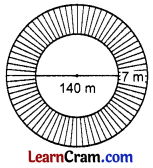# DAV Class 7 Maths Chapter 11 Worksheet 5 Solutions

The DAV Maths Class 7 Solutions and DAV Class 7 Maths Chapter 11 Worksheet 5 Solutions of Perimeter and Area offer comprehensive answers to textbook questions.

## DAV Class 7 Maths Ch 11 WS 5 Solutions

Question 1.
Find the circumference of a circle whose radius is 7 cm.
Circumference of a circle = 2πr
= 2 × $$\frac{22}{7}$$ × 7
= 44 cm
Hence the required circumference = 44 cm.

Question 2.
Find the diameter of a circle whose circumference is 66 m.
Circumference of a circle = 2πr
66 = 2 × $$\frac{22}{7}$$ × r
r = $$\frac{66 \times 7}{2 \times 22}=\frac{21}{2}$$ m
∴ Diameter = 2 × radius = 2× $$\frac{21}{2}$$ = 21 m
Hence the required diameter = 21 m

Question 3.
If circumference of a circle is 176 cm, find its radius.
Circumference of the circle = 2πr
176 = 2 × $$\frac{22}{7}$$ × r
∴ r = $$\frac{176 \times 7}{22 \times 2}$$ = 28 cm
Hence the required radius = 28 cm.Question 4.
The diameter of a wheel is 1.4 m, find its circumference.
Diameter = 1.4 m
∴ its radius = $$\frac{1.4}{2}$$ = 0.7 m
Circumference = 2πr = 2 × $$\frac{22}{7}$$ × 0.7 = 4.4 m
Hence the required circumference = 4.4 m.

Question 5.
The radii of two circles are in the ratio 2 : 3. What is the ratio of their circumference?
Let the radii of the two circles be 2x cm and 3x cm and C1 and C2 be their radii respectively.
∵ $$\frac{C_1}{C_2}=\frac{2 \pi r_1}{2 \pi r_2}$$
$$\frac{C_1}{C_2}=\frac{r_1}{r_2}=\frac{2 x}{3 x}=\frac{2}{3}$$
Hence the ratio of their circumference is 2 : 3.

Question 6.
Tire moon is nearly 385000 km away from the earth. It takes a round of the earth every month. How much distance does it travel in one month?
Radius of the circular path = 385000 km.
∴ Its circumference = 2πr
= 2 × $$\frac{22}{7}$$ × 385000
= 2 × 22 × 55000
= 2420000 km.
Hence the distance covered by moon in one month
= 2420000 km.

Question 7.
The diameter of the wheel of car is 35 cm. How much distance will it cover in 1000 revolutions?
The diameter of the wheel = 35 cm
∴ Its radius = $$\frac{35}{2}$$ cm
Circumference = 2πr
= 2 × $$\frac{22}{7} \times \frac{35}{2}$$ = 110 cm
∴ Distance covered by the wheel in 1000 revolutions
= 1000 × 110 cm
= 110000 cm = 1.1 km.
Hence the required distance covered = 1.1 km.

Question 8.
The radius of the wheel of a bus is 0.70 m. How many revolutions will it make in covering 22 km?
Circumference of the wheel = 2πr
= 2 × $$\frac{22}{7}$$ × 0.70 = 4.4 m
Hence the required number of revolutions = 5000 m.

Question 9.
A wire is in the form of a circle with radius 42 cm. It is bent into a square. Find the sides of the square.
Circumference of the circle = 2πr = 2 × $$\frac{22}{7}$$ × 42 = 264 cm
Perimeter of the square = 4 × side
Now 4 × side = 264
∴ Side = $$\frac{264}{4}$$ = 66 cm
Hence the side of the square = 66 cm.Question 10.
The diameter of a circular park is 140 m. Around it on the outside, a path having the width of 7 m is constructed. If the path has to be fenced from inside and outside at the rate of ₹ 7 per meter, find its total cost.Inner circumference = 2πr
= 2 × $$\frac{22}{7}$$ × 70
= 440 m
Radius of the outer park = 70 + 7 = 77 m
Outer circumference = 2π × r
= 2 × $$\frac{22}{7}$$ × 77 = 484 m
Total length of the two circumference
= 440 m + 484 m = 924 m
Cost of fencing = 7 × 924 = ₹ 6468
Hence the required cost = ₹ 6468.

Question 11.
An athlete runs around a circular park 10 times. If the diameter of park is 280 m, find the distance covered by the athlete in km.
Diameter of the circular park = 280 m
Its radius = $$\frac{280}{2}$$ = 140 m
Circumference = 2πr
= 2 × $$\frac{22}{7}$$ × 140 = 880 m
Total distance travelled by the athlete in 10 rounds = 880 × 10
= 8800 m
= 8.8 km.
Hence the required distance = 8.8 km.

Question 12.
A circular piece of the wire is converted into a rhombus of side 11 cm. Find the diameter of the circular piece.
Perimeter of the rhombus = 11 × 4 = 44 cm
∴ Circumference of the circular piece of wire = 44 cm
Circumference = 2πr
44 = 2 × $$\frac{22}{7}$$ × r
∴ r = $$\frac{44 \times 7}{2 \times 22}$$ = 7cm
∴ Its diameter = 2 × 7 = 14 cm
Hence the required diameter = 14 cm.

Question 13.
A race track is in the form of a ring whose inner circumference is 352 m, and the outer circumference is 396 m. Find the width of the track.
Let the radii of the outer and inner circles be r1 and r2 respectively.
∴ Inner circumference = 2πr2
352 = 2 × $$\frac{22}{7}$$ × r2
r2 = $$\frac{352 \times 7}{2 \times 22}$$ = 56 m
Outer circumference = 2πr1
396 = 2 × $$\frac{22}{7}$$ × r1
r1 = $$\frac{352 \times 7}{2 \times 22}$$ = 56 m
∴ Width of the track = r1 – r2 = 63 m – 56 m = 7 m
Hence the required width = 7 m.Question 14.
Radius of a circular region is 63 cm. Find the least length of rope which is sufficient to encircle the circular region.
Circumference = 2πr = 2 × $$\frac{22}{7}$$ × 63 = 396 cm